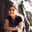a concise shot of dev knowledge
Become a Contributor
Concise shots of dev knowledge

RELATED TAGS

general
community creator

# What is a hash function?Anjana Shankar

In simple words, a hash function is a function that maps any arbitrary value to a certain fixed range.

For example, you may want to map the values from 1 to 100 within a range of 1 to 10. A simple way to do this would be to divide the range into 10 equal parts and map each to a single number. The diagram below demonstrates this:

Hash Functions are typically used in applications where data storage and retrieval requirements expect a constant time complexity. A good hash function is one that uniformly distributes data into different buckets.

## Cryptographic hash functions

Specialized hash functions that guarantee certain security properties are widely used in the field of cryptography and information security. For example, applications that need to verify the integrity of confidential data use a cryptographic hash function. Password verification and digital signature schemes also rely on such functions.

Such functions are often called one-way functions – it is practically infeasible to invert them, i.e., provided the output of the hash function, it should be infeasible to arrive at the input that produced the given output.

### Properties of cryptographic hash functions

1. Pre-image resistance
Reversing a hash function to obtain the original string should be impossible.
2. Weak-collision resistance
Given the input i0, it should be difficult to find another input i1, such that the hash of both values is the same.
3. Strong-collision resistance
It should be difficult to find two different messages with same hash value.
4. Determinism
The same string should generate the same hash every single time.
5. Non-Predictable
There should be no way to predict the hash from a password.
6. Diffusion
A small change in the original string should lead to a big change in the resulting hash.

## Popular Hash Functions

### Non-Cryptographic Hash Functions

#### 1.FNV

A slightly modified variant of FNV was used as the default hash function in Python.

It is widely used in zlib compression library.

### 1. RIPEMD

RIPEMD is used in Bitcoin and related applications.

#### 2. Scrypt

Scrypt is used as a password-based key derivation function for password hashing.

## Hash Functions applications

### 1. Object Equality.

In many programming languages, a hashcode of an object is used to determine if two objects are equal.

### 2. Bloom Filter

Hash functions are employed in order to quickly verify if the element is a member of the set.

### 3. Data Integrity

This is one of the most common applications of hash functions – it certifies to the user that the data is unchanged. However, it does not guarantee the originality of the file, so the attacker could potentially change both the file and the hash.

### 4. Password Storage and Verification

In the simplest verification scheme, the passwords are stored as a one way hash. When you enter your password, it is hashed and then the two hashes are compared to each other.

RELATED TAGS

general
community creator

CREATORAnjana Shankar
RELATED COURSES

View all Courses

Keep Exploring

Learn in-demand tech skills in half the time